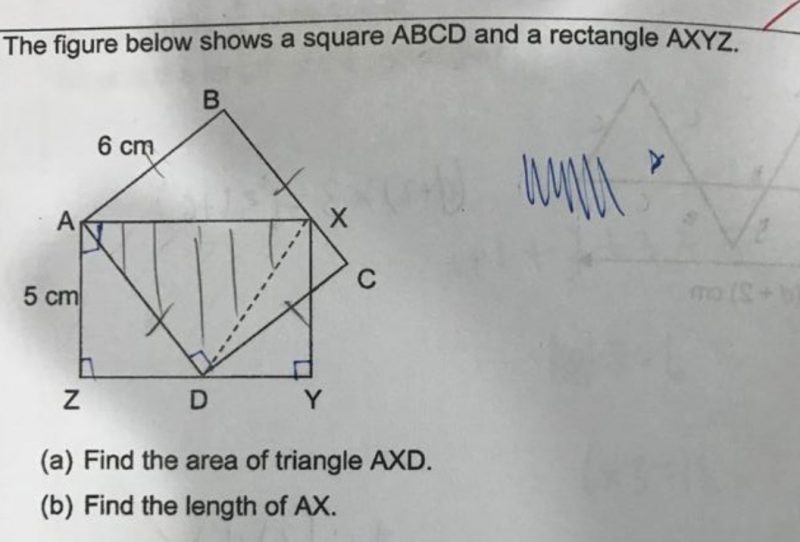# QuestionTeachers, pls help to solve. Thank you.

2 Answers

# Answer

The area of triangle AXD can be calculated directly as half the area of the square since the base is AD and the perpendicular height is AB.

½ × 6 × 6 = 18 cm²

0 Replies 0 Likes

(a) Area of Δ ABX + Area Δ DCX

= 0.5 x (BX) x 6 + 0.5 x (XC) x 6

= 3 (BX) + 3 (XC)

= 3 (BX + XC)  =  3 x 6   = 18 cm²

Area of Δ AXD

= Area of Square ABCD – (sum of Area of Δ ABX + Area Δ DCX )

= 36 – 18 = 18 cm²

(b) Area of Δ AXD   = 0.5 x AX x AZ

18  = 0.5 x AX x 5

AX = 7.2  cm²

0 Replies 0 Likes